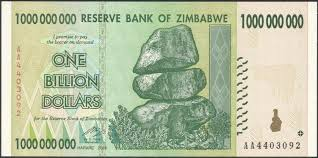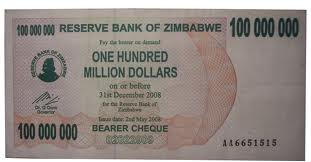# Difference between Billion and Million

##### Key Difference: Million is used to denote a number that can be expressed as 10^6 or 1000000, whereas Billion is expressed as 10^9 or 10000000000.

Numbers are fun to deal with, but when it comes to big numbers then we need some easy names to refer them. Billion and million are such words that create a picture of some large numbers. Yes, it is absolutely right that both represent large numbers. Million is used to denote a number that can be expressed as 10^6 or 1000000, whereas Billion is expressed as 10^9 or 10000000000.Million is a natural number that comes between 999,999 and 1,000,001. Billion lies between 999,999,999 and 1,000,000,000. The word ‘millian’ derived from Latin where 1000 was known as mille and hence, 1,000,000 started being referred as million, meaning a large thousand. Billion originated from French word bi- (“two”) + -illion, denoting a thousand million.It is easy to refer these large numbers with millions and billions instead of putting a figure with 6 or 9 zeroes. Another term that can be defined in the context of million and billions is trillions denoting 10^12 or 1000,000,000,000, meaning thousand billion. A person is known to be a millionaire if the assets owned by him are equal or more than a million. Similarly, a billionaire is the individual with assets equal or greater than a billion.

Image Courtesy: commons.wikimedia.org, toosoxy.wordpress.com